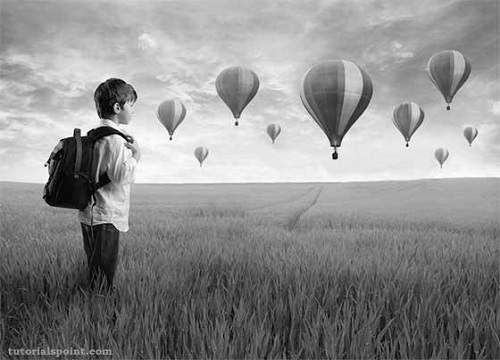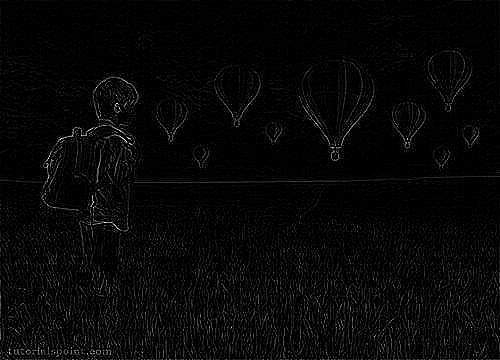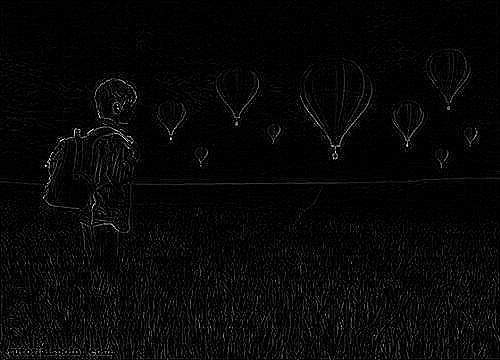# Java DIP - Laplacian Operator

Laplacian Operator is also a derivative operator which is used to find edges in an image. The major difference between Laplacian and other operators like Prewitt, Sobel, Robinson, and Kirsch is that these all are first order derivative masks but Laplacian is a second order derivative mask.

We use OpenCV function filter2D to apply Laplacian operator to images. It can be found under Imgproc package. Its syntax is given below −

```filter2D(src, dst, depth , kernel, anchor, delta, BORDER_DEFAULT );
```

The function arguments are described below −

Sr.No. Arguments
1

src

It is source image.

2

dst

It is destination image.

3

depth

It is the depth of dst. A negative value (such as -1) indicates that the depth is the same as the source.

4

kernel

It is the kernel to be scanned through the image.

5

anchor

It is the position of the anchor relative to its kernel. The location Point (-1, -1) indicates the center by default.

6

delta

It is a value to be added to each pixel during the convolution. By default it is 0.

7

BORDER_DEFAULT

We let this value by default.

Apart from the filter2D() method, there are other methods provided by the Imgproc class. They are described briefly −

Sr.No. Method & Description
1

cvtColor(Mat src, Mat dst, int code, int dstCn)

It converts an image from one color space to another.

2

dilate(Mat src, Mat dst, Mat kernel)

It dilates an image by using a specific structuring element.

3

equalizeHist(Mat src, Mat dst)

It equalizes the histogram of a grayscale image.

4

filter2D(Mat src, Mat dst, int depth, Mat kernel, Point anchor, double delta)

It convolves an image with the kernel.

5

GaussianBlur(Mat src, Mat dst, Size ksize, double sigmaX)

It blurs an image using a Gaussian filter.

6

integral(Mat src, Mat sum)

It calculates the integral of an image.

## Example

The following example demonstrates the use of Imgproc class to apply Laplacian operator to an image of Grayscale.

```import org.opencv.core.Core;
import org.opencv.core.CvType;
import org.opencv.core.Mat;

import org.opencv.highgui.Highgui;
import org.opencv.imgproc.Imgproc;

public class convolution {
public static void main( String[] args ) {

try {
int kernelSize = 9;

Mat destination = new Mat(source.rows(),source.cols(),source.type());

Mat kernel = new Mat(kernelSize,kernelSize, CvType.CV_32F) {
{
put(0,0,0);
put(0,1,-1)
put(0,2,0);

put(1,0-1);
put(1,1,4);
put(1,2,-1);

put(2,0,0);
put(2,1,-1);
put(2,2,0);
}
};

Imgproc.filter2D(source, destination, -1, kernel);
Highgui.imwrite("output.jpg", destination);

} catch (Exception e) {
System.out.println("Error: " + e.getMessage());
}
}
}
```

## Output

When you execute the given code, the following output is seen −

### Original ImageThis original image is convolved with the Laplacian Negative operator as given below −

### Laplacian Negative

 0 -1 0 -1 4 -1 0 -1 0

### Convolved Image(Laplacian Negative)This original image is convolved with the Laplacian Positive operator as given below −

### Laplacian Positive

 0 1 0 1 -4 1 0 1 0

### Convolved Image (Laplacian Positive)